# Luna Tech

Tutorials For Dummies.

# 0. 前言

Reference: 手把手带你刷二叉树（第一期） - labuladong 的算法小抄 (gitbook.io)

## 为什么要先刷二叉树？

/* 二叉树遍历框架 */
void traverse(TreeNode root) {
// 前序遍历（在调用所有递归function之前）
traverse(root.left)
// 中序遍历（在调用了一个递归function之后）
traverse(root.right)
// 后序遍历（在调用了所有递归function之后）
}


• 线性 - while/for 迭代
• 非线性 - recursive 递归

## 相关题目

226. 翻转二叉树 - 力扣（LeetCode） (leetcode-cn.com)

114. 二叉树展开为链表 - 力扣（LeetCode） (leetcode-cn.com)

116. 填充每个节点的下一个右侧节点指针 - 力扣（LeetCode） (leetcode-cn.com)

# 1. 二叉树递归遍历框架的重要性

• 快速排序是二叉树的前序遍历；
• 归并排序是二叉树的后序遍历；

## 快速排序的代码框架

• 对一个 number array nums[lo..hi]进行排序，先找到一个 p 元素作为分界点；
• 交换元素，使得 nums[lo...p-1] 小于等于 nums[p]nums[p+1..hi] 大于 nums[p]
• 递归思维，继续在 nums[lo...p-1]nums[p+1..hi] 里面分别找新的分界元素 p'，然后继续进行元素交换

void sort(int[] nums, int lo, int hi) {
/********************** 前序遍历位置 *********************/
/* 假定我们有个 partition function，通过交换元素构建分界点 p */
int p = partition(nums, lo, hi);
/*******************************************************/

sort(nums, lo, p - 1);
sort(nums, p + 1, hi);
}


## 归并排序的代码框架

• nums[lo..hi] 进行排序，先找到中点，对 nums[lo..mid] 排序，再对 nums[mid+1..hi] 排序
• 最后把这两个有序的子数组合并，整个数组就排好序了
void sort(int[] nums, int lo, int hi) {
int mid = (lo + hi) / 2;
sort(nums, lo, mid);
sort(nums, mid + 1, hi);

/****** 后序遍历位置 ******/
/* 合并两个排好序的子数组  */
merge(nums, lo, mid, hi);
/************************/
}


# 2. 写递归算法的秘诀

## 举例：计算二叉树一共有几个节点

// 定义：count(root) 返回以 root 为根的树有多少节点
int count(TreeNode root) {
// base case
if (root == null) return 0;
// 自己加上子树的节点数就是整棵树的节点数
return 1 + count(root.left) + count(root.right);
}


• 该做什么 = 实现题目所要求的，比如这里就是计算总节点数 1 + count(root.left) + count(root.right)

• 什么时候做 = 判断代码的位置，到底是前序、中序还是后序。

# 3. 题目实战

## 226. 翻转二叉树

226. 翻转二叉树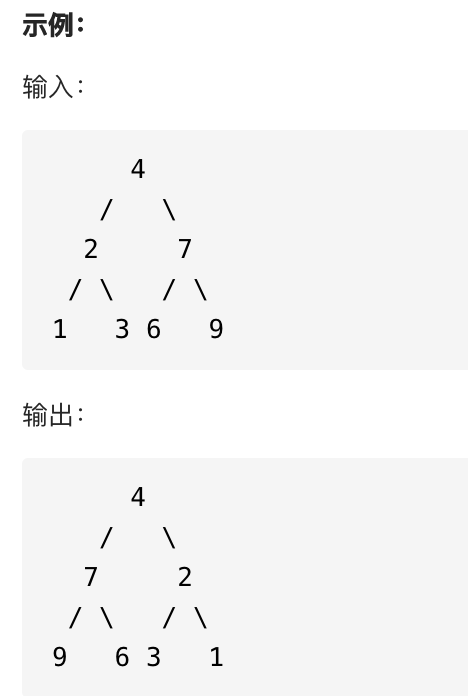### 代码

/**
* Definition for a binary tree node.
* public class TreeNode {
*     int val;
*     TreeNode left;
*     TreeNode right;
*     TreeNode() {}
*     TreeNode(int val) { this.val = val; }
*     TreeNode(int val, TreeNode left, TreeNode right) {
*         this.val = val;
*         this.left = left;
*         this.right = right;
*     }
* }
*/
class Solution {
public TreeNode invertTree(TreeNode root) {
// 特殊 case
if (root == null)
return null;
// 前序遍历，左右互换
TreeNode temp = root.left;
root.left = root.right;
root.right = temp;
// 进入递归，左右子树重复操作
invertTree(root.left);
invertTree(root.right);
// 返回结果
return root;
}
}


### 其他解法

class Solution {
public TreeNode invertTree(TreeNode root) {
// 特殊 case
if (root == null)
return null;
// 进入递归，左右子树重复操作
invertTree(root.left);
invertTree(root.right);
// 后序遍历，左右互换
TreeNode temp = root.left;
root.left = root.right;
root.right = temp;
// 返回结果
return root;
}
}


// not working
class Solution {
public TreeNode invertTree(TreeNode root) {
// 特殊 case
if (root == null)
return null;
// 进入递归，左子树重复操作
invertTree(root.left);
// 中序遍历，左右互换
TreeNode temp = root.left;
root.left = root.right;
root.right = temp;
// 进入递归，右子树重复操作
invertTree(root.right);

// 返回结果
return root;
}
}


class Solution {
public TreeNode invertTree(TreeNode root) {
// 特殊 case
if (root == null)
return null;
// 进入递归，左子树重复操作
invertTree(root.left);
// 中序遍历，左右互换
TreeNode temp = root.left;
root.left = root.right;
root.right = temp;
// 进入递归，右子树重复操作
invertTree(root.left); // 注意这里的 node 实际上是之前的 right
// 返回结果
return root;
}
}


## 116. 填充每个节点的下一个右侧节点指针

116. 填充每个节点的下一个右侧节点指针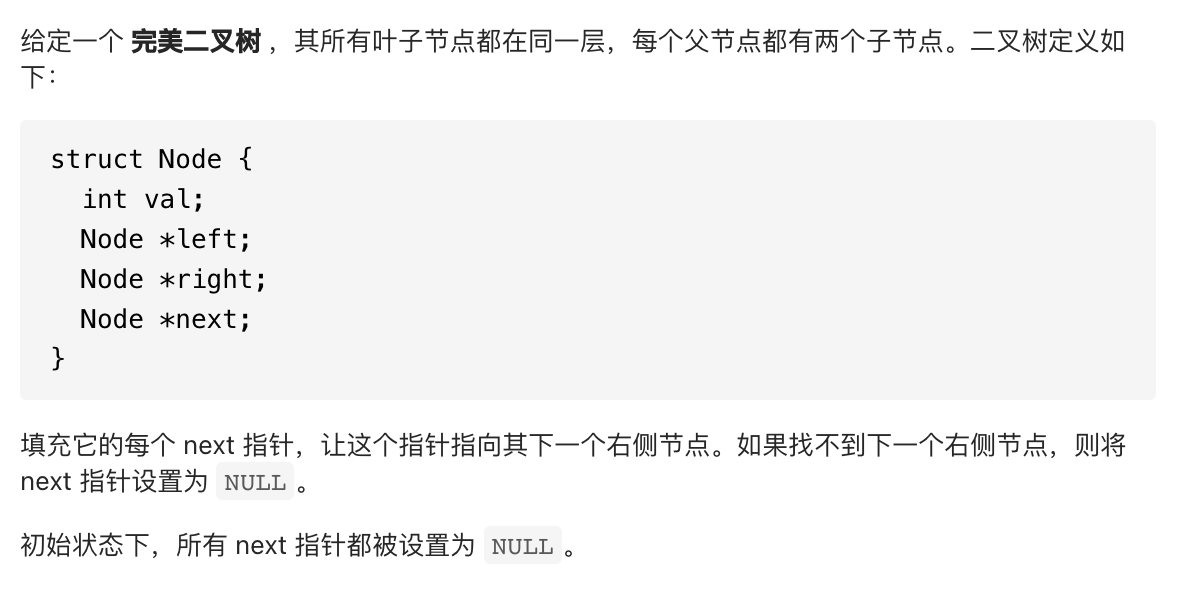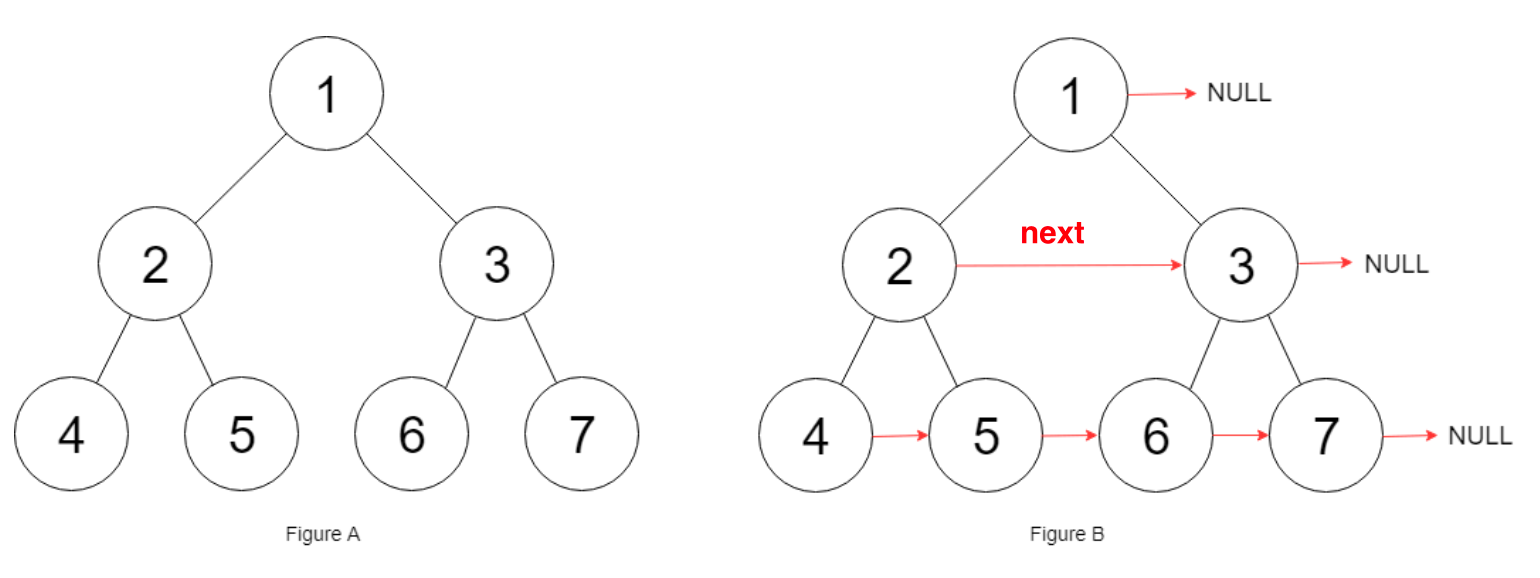root.left.next = root.right; 就是我们的核心逻辑；

/*
// Definition for a Node.
class Node {
public int val;
public Node left;
public Node right;
public Node next;

public Node() {}

public Node(int _val) {
val = _val;
}

public Node(int _val, Node _left, Node _right, Node _next) {
val = _val;
left = _left;
right = _right;
next = _next;
}
};
*/

class Solution {
public Node connect(Node root) {
if (root == null || root.left == null) {
return root;
}
root.left.next = root.right;
connect(root.left);
connect(root.right);
return root;
}
}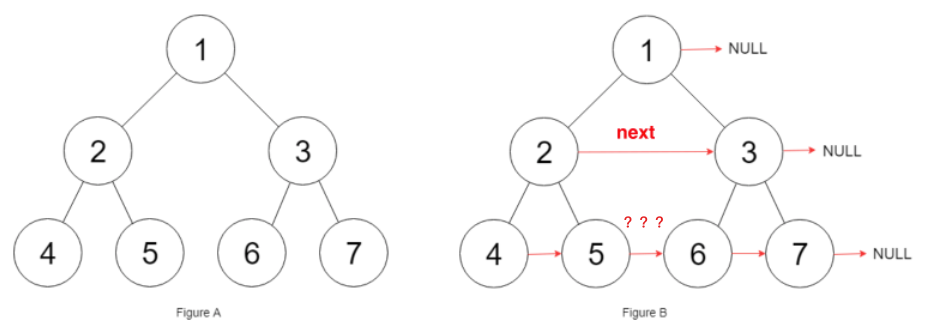/*
// Definition for a Node.
class Node {
public int val;
public Node left;
public Node right;
public Node next;

public Node() {}

public Node(int _val) {
val = _val;
}

public Node(int _val, Node _left, Node _right, Node _next) {
val = _val;
left = _left;
right = _right;
next = _next;
}
};
*/

class Solution {
// 主函数
public Node connect(Node root) {
if (root == null) return null;
connectTwoNode(root.left, root.right);
return root;
}

// 辅助函数
void connectTwoNode(Node node1, Node node2) {
if (node1 == null || node2 == null) {
return;
}
/**** 前序遍历位置 ****/
// 将传入的两个节点连接
node1.next = node2;

// 连接相同父节点的两个子节点
connectTwoNode(node1.left, node1.right);
connectTwoNode(node2.left, node2.right);
// 连接跨越父节点的两个子节点
connectTwoNode(node1.right, node2.left);
}
}


## 114. 二叉树展开为链表

114. 二叉树展开为链表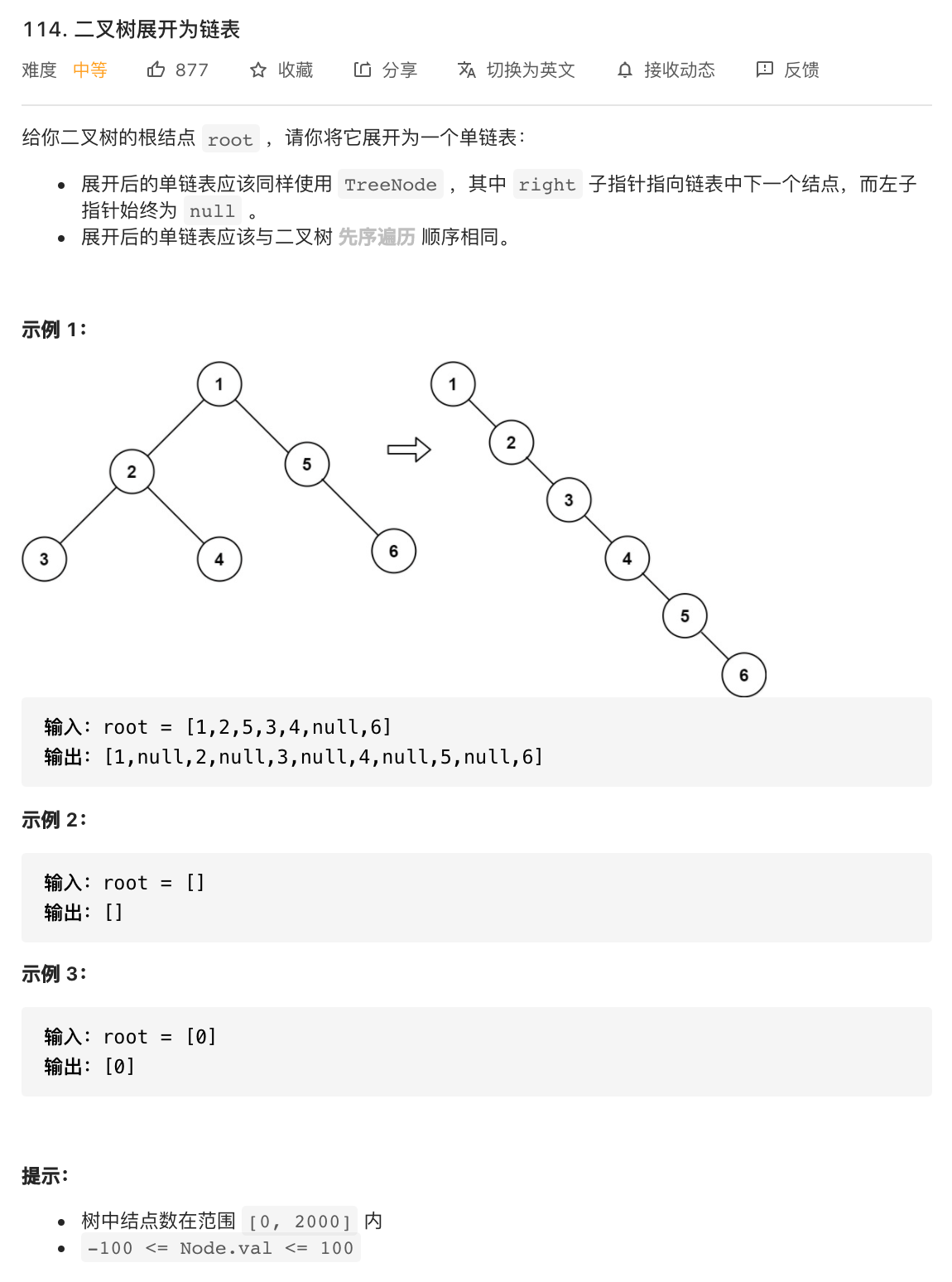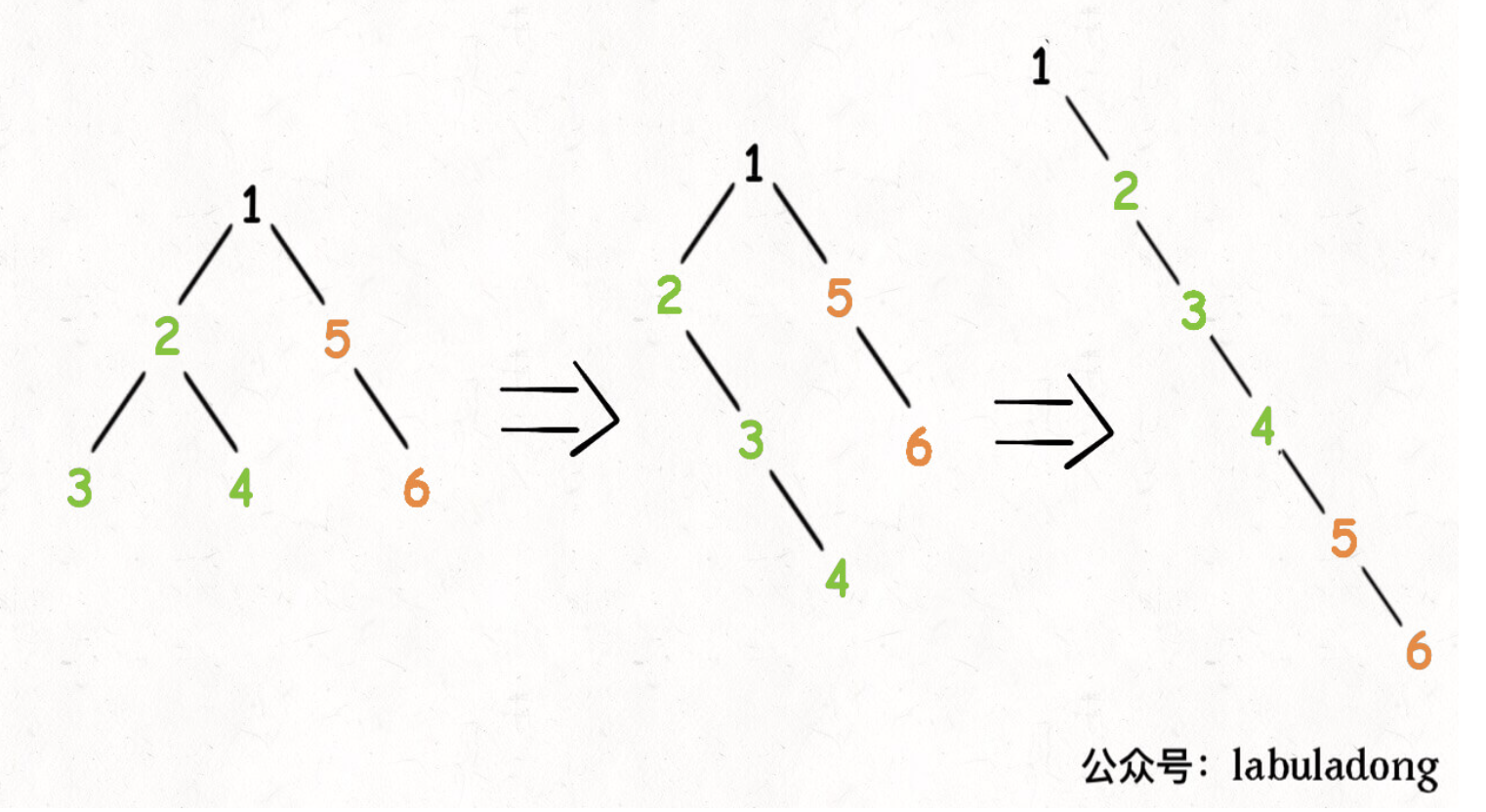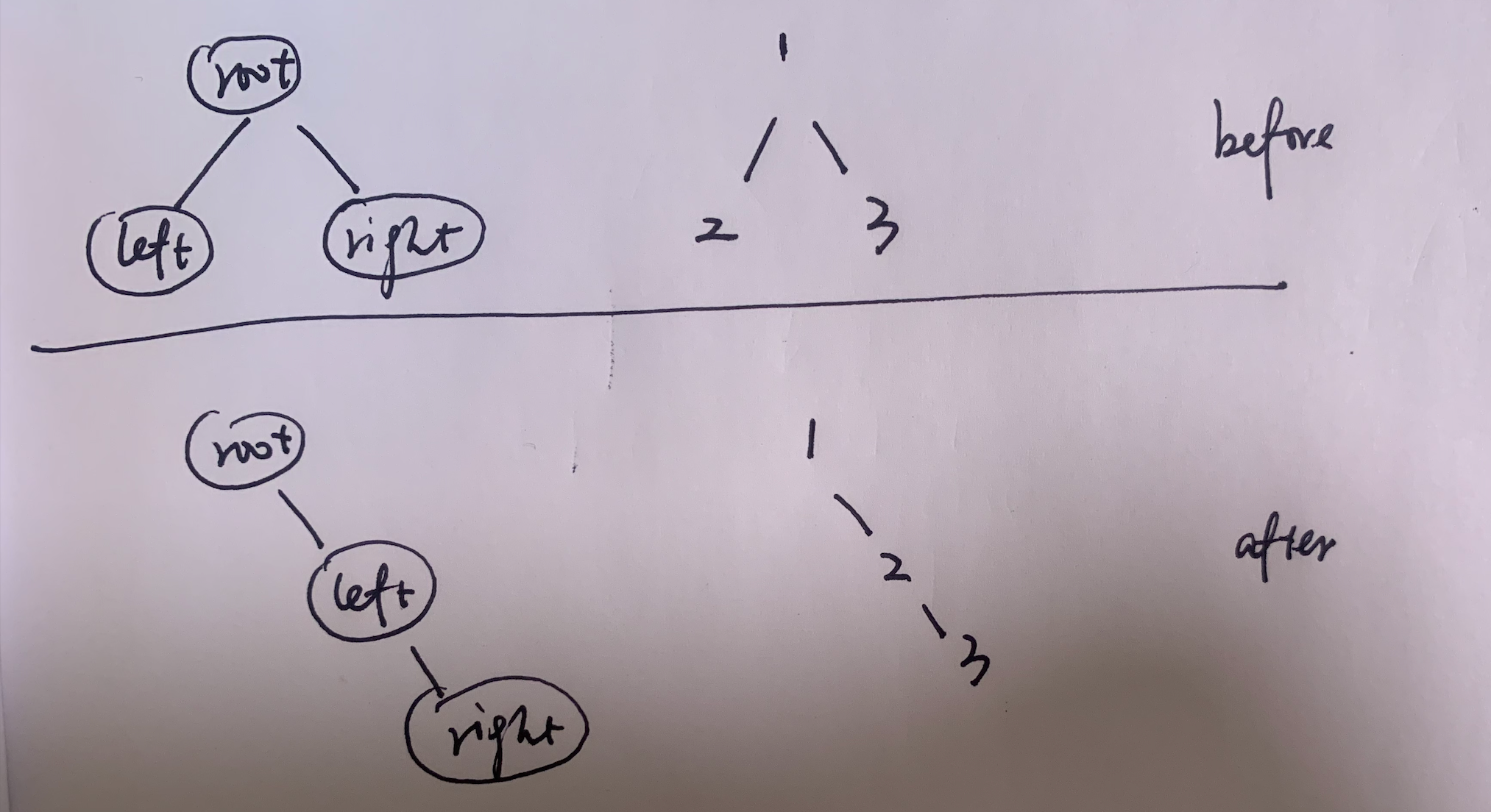TreeNode orgRight = root.right;
root.right = null;
root.right = root.left;
root.right.right = orgRight;


PS: 题目给的 method 是 void type，所以我们不需要 return root，只需要 return 就可以了（有点绕）。

/**
* Definition for a binary tree node.
* public class TreeNode {
*     int val;
*     TreeNode left;
*     TreeNode right;
*     TreeNode() {}
*     TreeNode(int val) { this.val = val; }
*     TreeNode(int val, TreeNode left, TreeNode right) {
*         this.val = val;
*         this.left = left;
*         this.right = right;
*     }
* }
*/
class Solution {
// 定义：将以 root 为根的树拉平为链表
public void flatten(TreeNode root) {
// base case, 根据题目示例2来写
if (root == null) return;
// flatten both left and right trees
flatten(root.left);
flatten(root.right);

/**** 后序遍历位置 ****/
// 1、左右子树已经被拉平成一条链表
TreeNode left = root.left;
TreeNode right = root.right;

// 2、将左子树作为右子树
root.left = null;
root.right = left;

// 3、将原先的右子树接到当前右子树的末端
TreeNode p = root;
while (p.right != null) {
p = p.right;
}
p.right = right;
}
}


### 为什么要做后序遍历呢？

class Solution {
public void flatten(TreeNode root) {
// base case
if (root == null) return;

/**** 前序遍历 ****/
// 1、储存最初的左右节点
TreeNode left = root.left;
TreeNode right = root.right;

// 2、进行第一步 flatten 操作（左子树）
root.left = null;
root.right = left;

// 3、进行第二步 flatten 操作（右子树）
TreeNode p = root;
while (p.right != null) {
p = p.right;
}
p.right = right;

// 调用递归（使用未经改变的两个节点）
flatten(left);
flatten(right);
}
}